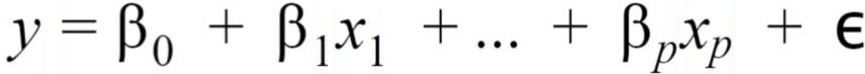# Multivariate analysis

Modified on Mon, 23 Nov 2020 at 11:06 AM

# What is a multivariate analysis?

In biomedical research, an usual goal is to assess the effect of environmental, psycho-social or medical factors on the occurrence, the severity, the diagnosis, the evolution or the recovery of a disease.

To do this, one could perform a multivariate analysis which studies the relationship between more than two variables: a variable, called variable to explain, is wished to be explained by a set of other variables, called explanatory variables.

This relation is mathematically express as:where y is the response variable, x1 to xp are the explanatory variables, β1 to βp are the coefficients of the explanatory variables, β0 is the intercept and Ɛ is the error term (or residuals term).

In EMS, two kinds of regression are available: the linear regression which allows to study a numeric response variable and the logistic regression which allows to study a binary variable (categorical variable with two modalities as yes/no variables).

# In which cases could it be interesting to compute a multivariate analysis?

A multivariate analysis is interesting for example:

• To identify variables which can be risk factors of a disease or risk factors of a complication
• To predict the parameters that affect a clinical score or the level of pain

Let's take an example. You want to prove that a new medication decreases the risk of myocardial infractions. In addition to the drug you test, you need to take into account simultaneously known risk factors for myocardial infractions. The response variable will be Presence of myocardial infraction (yes/no) and the explanatory variables would be: the drug you test (yes/no), age (in years), sex (women, men), smoking status (non smoker, former smoker, smoker), alcohol consumption, nutrition diet (omnivorous, vegetarian, Mediterranean), history of cardiovascular diseases (yes/no). The model will be mathematically written as:

Presence of myocardial infraction = β0 + β1 drug + β2 age + β3 sex + β4.1 smoking status=smoker  + β4.2 smoking status=former smoker + βalcohol consumption + β6.1 nutrition diet=vegetarian + + β6.2 nutrition diet=Mediterranean + βhistory of cardiovascular diseases + Ɛ.

# How should I interpret the results of my multivariate analysis?

In linear regression, the coefficients of a given explanatory variable indicates of how many points the response variable increases when the explanatory variables increase by 1 point, the other variables being fixed. For instance, if the coefficient of Age is 0.3 in the model used to explain the variable Number of fractures in the past 12 months, that means that for each increase of 1 year on the variable Age, the variable Number of fractures in the past 12 months increases by 0.3. In the same model, the coefficient of sex=Female is 0.8, meaning that the variable Number of fractures in the past 12 months is increases by 0.8 for sex=Women compared to the modality sex=Male.

In logistic regression, because of the transformation of the response variable with the logit function, the coefficients are less easy to understand, the odds ratio are more usually used.

For instance, if the coefficient of Age is 0.5 in the model used to explain the variable Diagnosis of cancer (yes/no), that means that for each increase of 1 year on the variable Age, the log-odds of the variable Diagnosis of cancer increases by 0.5. Exponentiating the coefficient, the odds-ratio of the variable Age is 1.65, meaning that when the Age increases by 1 year, the odds of having a positive diagnosis of cancer increases by 65%. In the same model, the coefficient of sex=Female is 0.2, meaning that the log-odds of having a positive diagnosis of cancer when being a woman increases by 0.2 compared to the log-odds when being a man, so the odds of having a positive diagnosis of cancer when being a woman is 1.22 meaning that the odds is increased by 22 %.

The coefficient of intercept has not usually a sens and should not be interpreted.